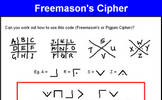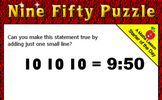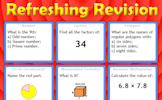# Non-linear graphs

### Term 1 starting in week 3 :: Estimated time: 2 weeks

• Plot and read from cubic graphs
• Plot and read from reciprocal graphs
• Recognise graph shapes
• Identify and interpret roots and intercepts of quadratics

For higher-attaining pupils:

• Understand and use exponential graphs
• Find and use the equation of a circle centre (O, O)
• Find the equation of the tangent to any curve

This page should remember your ticks from one visit to the next for a period of time. It does this by using Local Storage so the information is saved only on the computer you are working on right now.

## Lesson Starters

Here are some suggestions for whole-class, projectable resources which can be used at the beginnings of each lesson in this block.

### 1st Lesson#### Two Twins and Tim

A tricky problem set on a coordinate grid.

### 2nd Lesson#### Four Factors

Find four single digit numbers that multiply together to give 120. How many different ways are there of answering this question?

### 3rd Lesson#### Freemason's Cipher

Find symmetric words in this ancient cipher.

### 4th Lesson#### Dice Reflections

A dice is reflected in two mirrors. What numbers can be seen?

### 5th Lesson### 6th Lesson#### Refreshing Revision

It is called Refreshing Revision because every time you refresh the page you get different revision questions.

Some of the Starters above are to reinforce concepts learnt, others are to introduce new ideas while others are on unrelated topics designed for retrieval practice or and opportunity to develop problem-solving skills.

White Rose ResourcesEnd of block assessments provide a quick progress check at the end of each block of learning to make sure students have understood the content covered. This Scheme of Learning was produced by White Rose Maths and is used here with permission granted on 30th June 2021.For All: